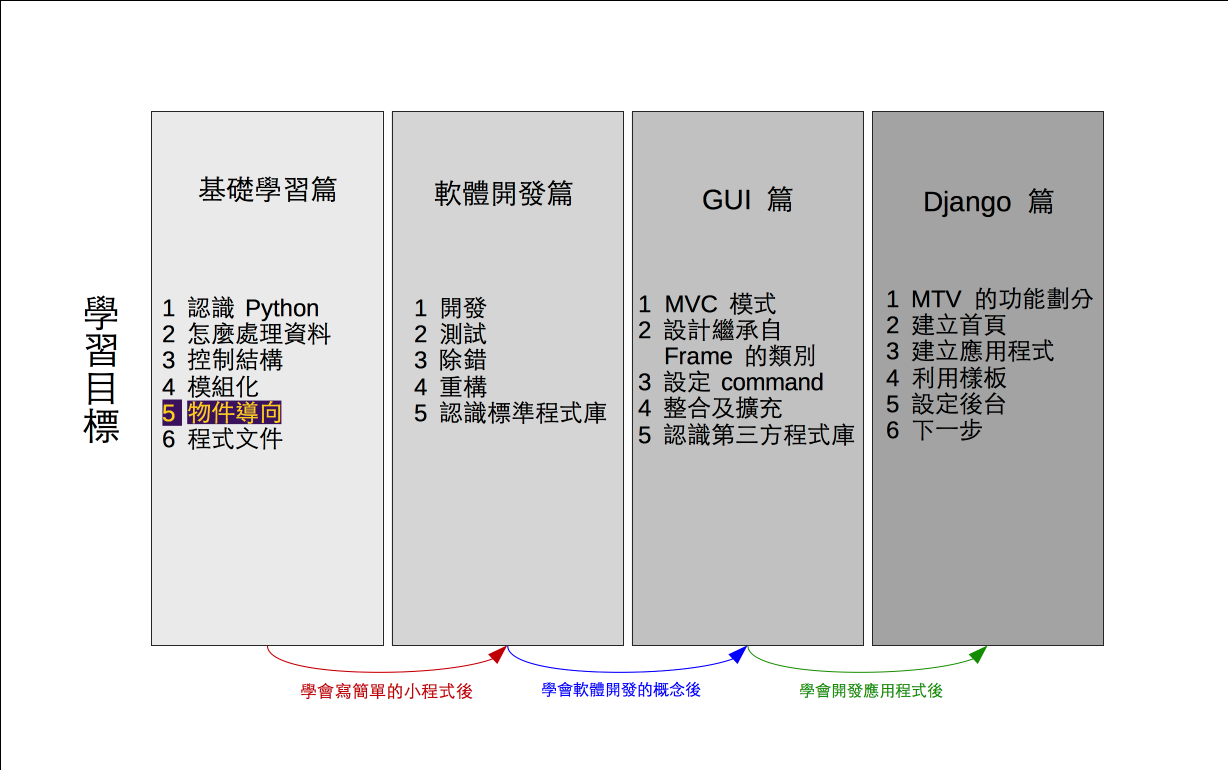# 單元 10 - 物件導向與封裝

～～學習進度表～～Encapsulation
Inheritance
Polymorphism

``````class Demo:
def set_att(self, a=22, b=33):
self.a = a
self.b = b

def do_something(self):
return self.a + self.b

d = Demo()
d.set_att()
print(d.do_something())
d.a = 5
print(d.do_something())

#《程式語言教學誌》的範例程式
# http://kaiching.org/
# 檔名：class_demo4.py
# 功能：示範 Python 中的類別
# 作者：張凱慶 */``````

``d.a = 5``

 \$ python class_demo4.py 55 38 \$

``````class Demo:
def set_att(self, a=22, b=33):
self.__a = a
self.__b = b

def do_something(self):
return self.__a + self.__b

d = Demo()
d.set_att()
print(d.do_something())
d.__a = 5
print(d.__a)
print(d.do_something())

#《程式語言教學誌》的範例程式
# http://kaiching.org/
# 檔名：class_demo5.py
# 功能：示範 Python 中的類別
# 作者：張凱慶 */``````

set_att() 中兩個實體屬性都已加上兩個底線

``````def set_att(self, a=22, b=33):
self.__a = a
self.__b = b``````

``````d.__a = 5
print(d.__a)``````

 \$ python class_demo5.py 55 5 55 \$

__a 重新被設定為 5 也印出 5 來，可是 do_something()回傳值仍是 55 ，程式順利執行結束沒有出錯，這是為什麼呢？

1. 物件導向程式設計有封裝、繼承及多型等三大基本特性。
2. 繼承像是親屬的重直關係（父母子女），多型則像是親屬的平行關係（兄弟姊妹）。
3. 封裝連帶的觀念就是資訊隱藏，類別的屬性只能在類別中處理，要在實體屬性前加上兩個斜線。
4. 外界要存取封裝過的實體屬性要透過 getter 方法，或用 setter 方法進行設定。

1. 物件導向程式設計有哪三大基本特性？
2. 什麼是封裝？為什麼要做封裝？
3. 什麼是繼承？繼承機制有什麼優點？
4. 什麼是多型？可以用什麼方式比擬嗎？

1. 寫一個程式 exercise1001.py ，利用 exercise0905.py 設計的 IntegerDemo ，封裝實體屬性 value
2. 寫一個程式 exercise1002.py ，利用 exercise0906.py 計算階層值的類別，封裝實體屬性 value
3. 寫一個程式 exercise1003.py ，利用 exercise0907.py 計算費氏數列的類別，封裝實體屬性 value